# Probabilistic Belief States and Bayesian Networks Where we

• Slides: 41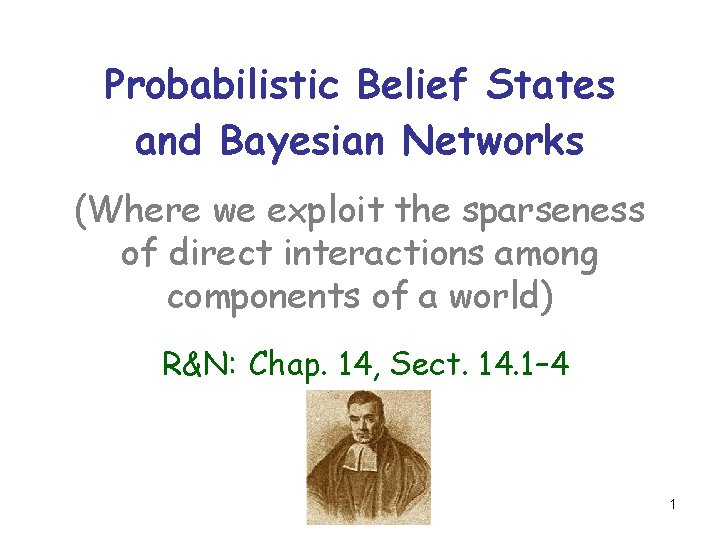Probabilistic Belief States and Bayesian Networks (Where we exploit the sparseness of direct interactions among components of a world) R&N: Chap. 14, Sect. 14. 1– 4 1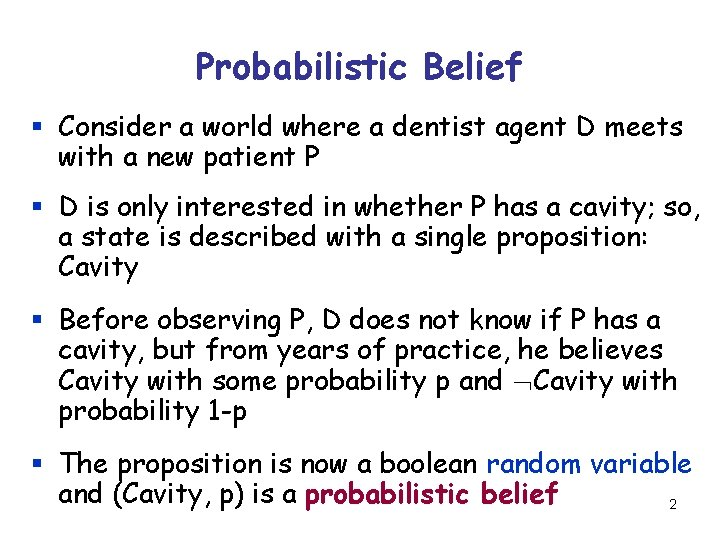Probabilistic Belief § Consider a world where a dentist agent D meets with a new patient P § D is only interested in whether P has a cavity; so, a state is described with a single proposition: Cavity § Before observing P, D does not know if P has a cavity, but from years of practice, he believes Cavity with some probability p and Cavity with probability 1 -p § The proposition is now a boolean random variable and (Cavity, p) is a probabilistic belief 2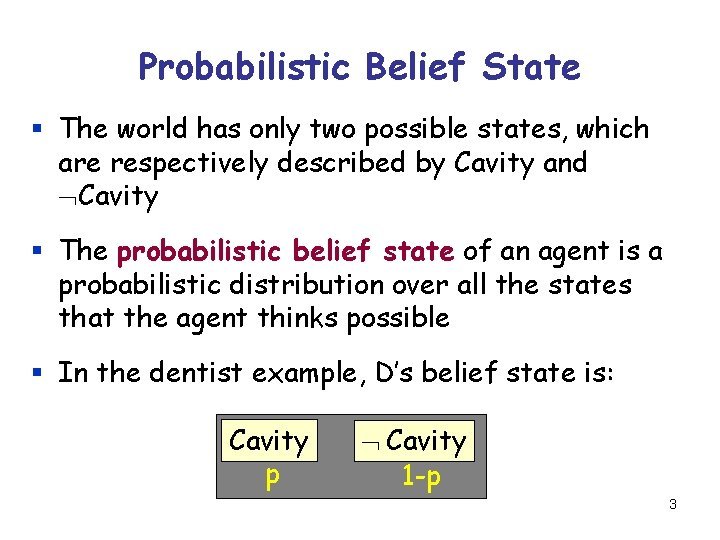Probabilistic Belief State § The world has only two possible states, which are respectively described by Cavity and Cavity § The probabilistic belief state of an agent is a probabilistic distribution over all the states that the agent thinks possible § In the dentist example, D’s belief state is: Cavity p Cavity 1 -p 3Vacuum Robot If the robot has no idea what the state of the world is, and thinks that all states are equally probable (using the “principle of indifference”), its belief state is: 1/8 1/8 4How are beliefs and belief states related? 1/16 1/4 1/8 1/16 1/4 (Clean(R 1), 5/16) (Clean(R 2), 0. 5) (In(Robot, R 1), 0. 5) (In(Robot, R 2), 0. 5) How a belief affects the entire belief state and the other beliefs? It is usually more convenient to deal with individual beliefs than with entire belief states, e. g. : - The robot may choose to execute Suck(R 2) only if Clean(R 2) has low probability - The robot may directly observe whether 5 Clean(R 1) or Clean(R 2)Back to the dentist example. . . § We now represent the world of the dentist D using three propositions – Cavity, Toothache, and PCatch § D’s belief state consists of 23 = 8 states each with some probability: {Cavity Toothache PCatch, . . . } 6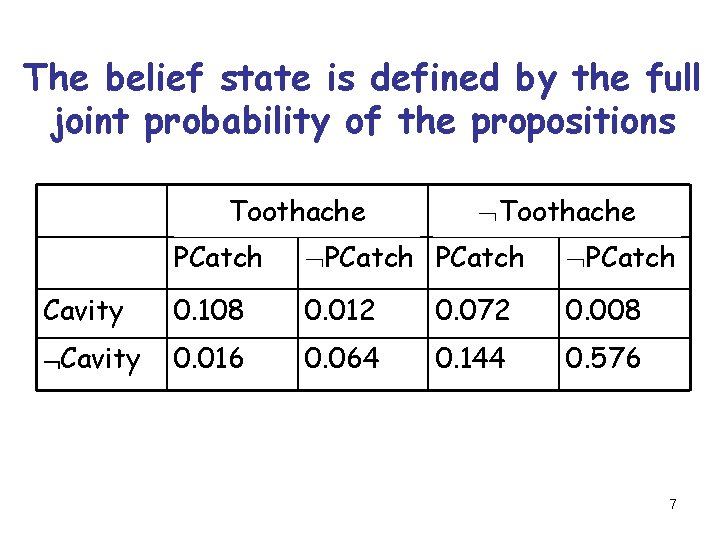The belief state is defined by the full joint probability of the propositions Toothache PCatch Cavity 0. 108 0. 012 0. 072 0. 008 Cavity 0. 016 0. 064 0. 144 0. 576 7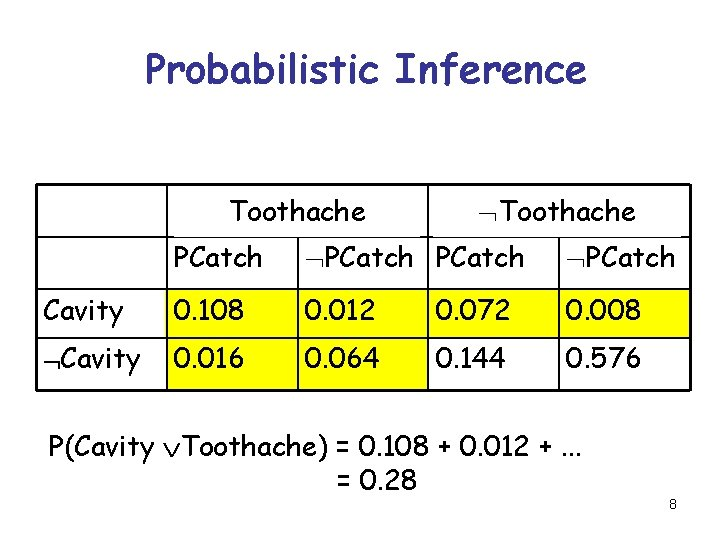Probabilistic Inference Toothache PCatch Cavity 0. 108 0. 012 0. 072 0. 008 Cavity 0. 016 0. 064 0. 144 0. 576 P(Cavity Toothache) = 0. 108 + 0. 012 +. . . = 0. 28 8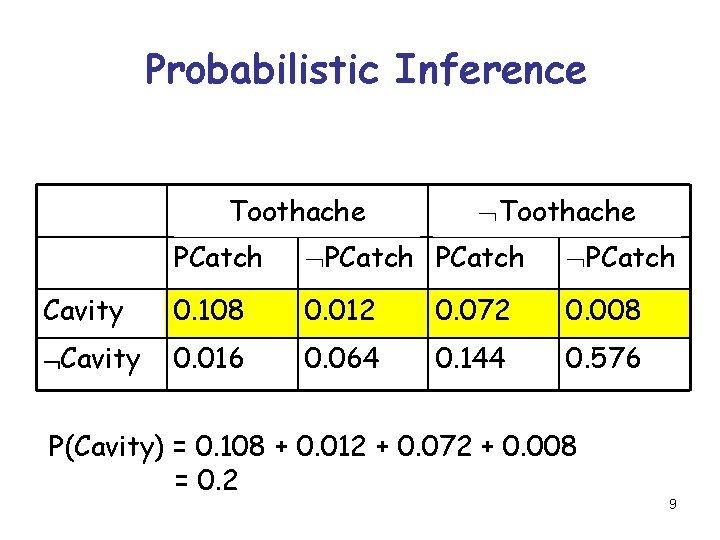Probabilistic Inference Toothache PCatch Cavity 0. 108 0. 012 0. 072 0. 008 Cavity 0. 016 0. 064 0. 144 0. 576 P(Cavity) = 0. 108 + 0. 012 + 0. 072 + 0. 008 = 0. 2 9Probabilistic Inference Toothache PCatch Cavity 0. 108 0. 012 0. 072 0. 008 Cavity 0. 016 0. 064 0. 144 0. 576 Marginalization: P(c) = St. Spc P(c t pc) using the conventions that c = Cavity or Cavity and that St is the sum over t = {Toothache, Toothache} 10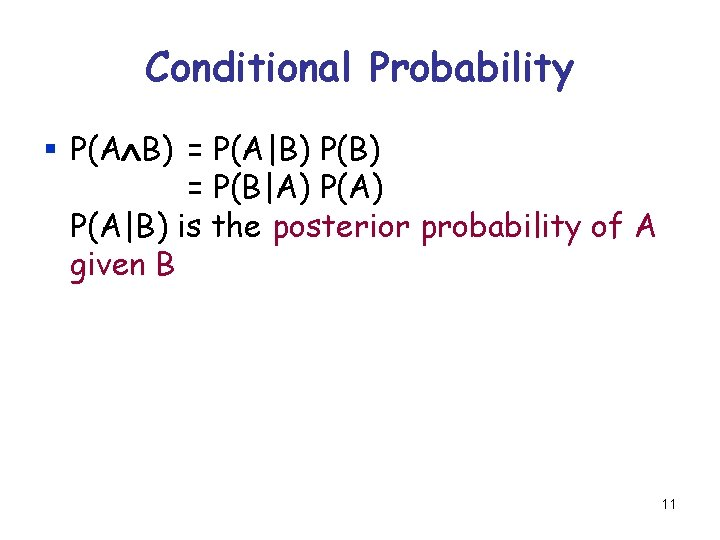Conditional Probability § P(A B) = P(A|B) P(B) = P(B|A) P(A|B) is the posterior probability of A given B 11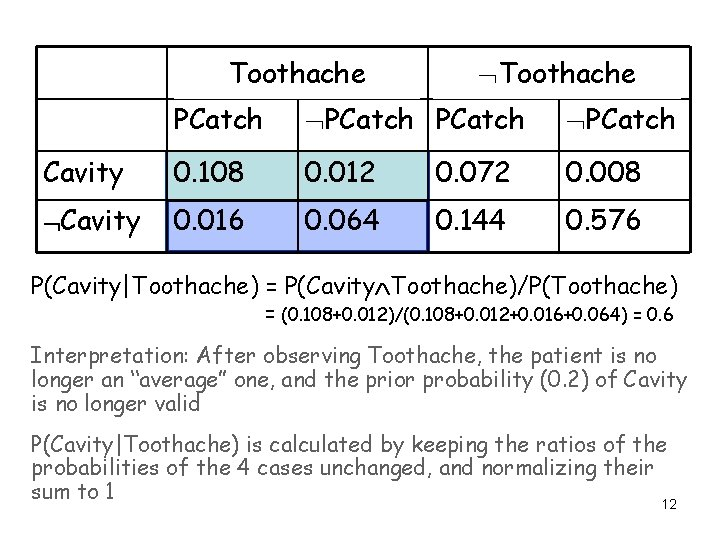Toothache PCatch Cavity 0. 108 0. 012 0. 072 0. 008 Cavity 0. 016 0. 064 0. 144 0. 576 P(Cavity|Toothache) = P(Cavity Toothache)/P(Toothache) = (0. 108+0. 012)/(0. 108+0. 012+0. 016+0. 064) = 0. 6 Interpretation: After observing Toothache, the patient is no longer an “average” one, and the prior probability (0. 2) of Cavity is no longer valid P(Cavity|Toothache) is calculated by keeping the ratios of the probabilities of the 4 cases unchanged, and normalizing their sum to 1 12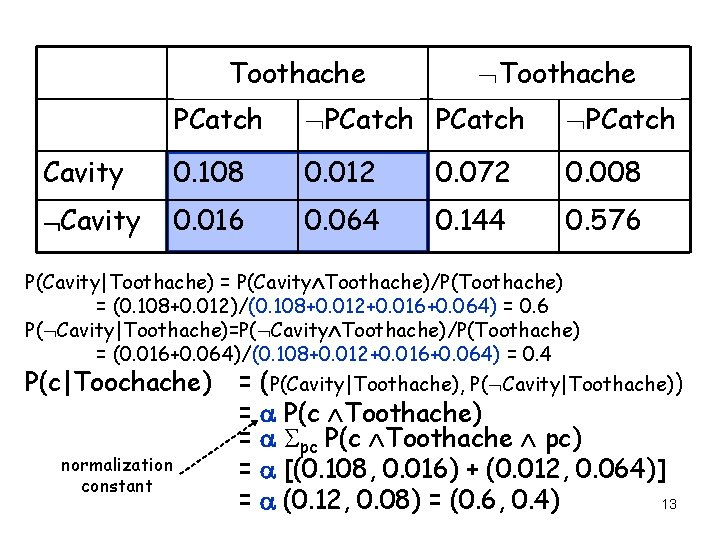Toothache PCatch Cavity 0. 108 0. 012 0. 072 0. 008 Cavity 0. 016 0. 064 0. 144 0. 576 P(Cavity|Toothache) = P(Cavity Toothache)/P(Toothache) = (0. 108+0. 012)/(0. 108+0. 012+0. 016+0. 064) = 0. 6 P( Cavity|Toothache)=P( Cavity Toothache)/P(Toothache) = (0. 016+0. 064)/(0. 108+0. 012+0. 016+0. 064) = 0. 4 P(c|Toochache) = (P(Cavity|Toothache), P( Cavity|Toothache)) normalization constant = a P(c Toothache) = a Spc P(c Toothache pc) = a [(0. 108, 0. 016) + (0. 012, 0. 064)] 13 = a (0. 12, 0. 08) = (0. 6, 0. 4)Conditional Probability § P(A B) = P(A|B) P(B) = P(B|A) P(A) § P(A B C) = P(A|B, C) P(B|C) P(C) § P(Cavity) = St. Spc P(Cavity t pc) = St. Spc P(Cavity|t, pc) P(t pc) § P(c) = St. Spc P(c t pc) = St. Spc P(c|t, pc)P(t pc) 14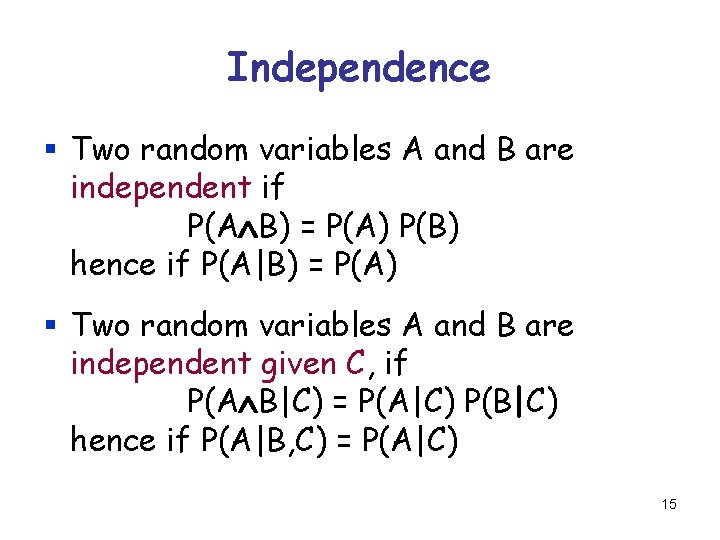Independence § Two random variables A and B are independent if P(A B) = P(A) P(B) hence if P(A|B) = P(A) § Two random variables A and B are independent given C, if P(A B|C) = P(A|C) P(B|C) hence if P(A|B, C) = P(A|C) 15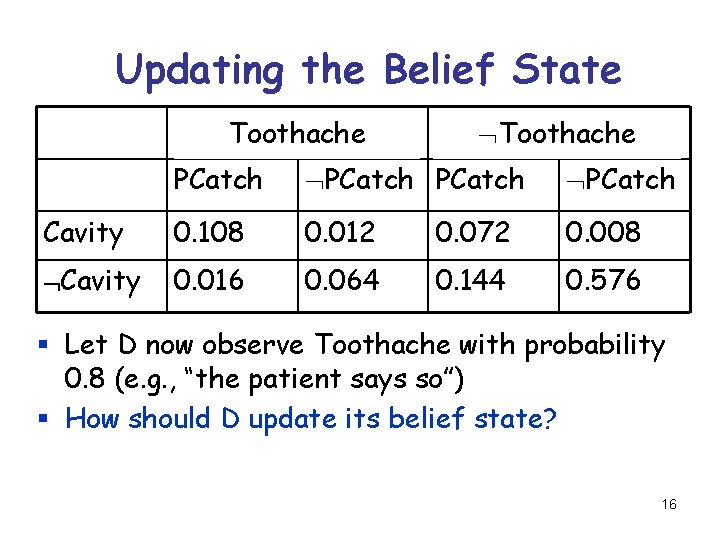Updating the Belief State Toothache PCatch Cavity 0. 108 0. 012 0. 072 0. 008 Cavity 0. 016 0. 064 0. 144 0. 576 § Let D now observe Toothache with probability 0. 8 (e. g. , “the patient says so”) § How should D update its belief state? 16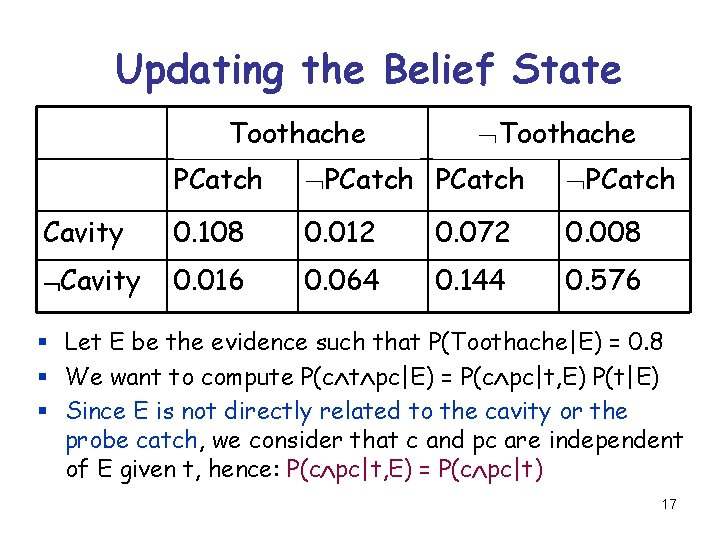Updating the Belief State Toothache PCatch Cavity 0. 108 0. 012 0. 072 0. 008 Cavity 0. 016 0. 064 0. 144 0. 576 § Let E be the evidence such that P(Toothache|E) = 0. 8 § We want to compute P(c t pc|E) = P(c pc|t, E) P(t|E) § Since E is not directly related to the cavity or the probe catch, we consider that c and pc are independent of E given t, hence: P(c pc|t, E) = P(c pc|t) 17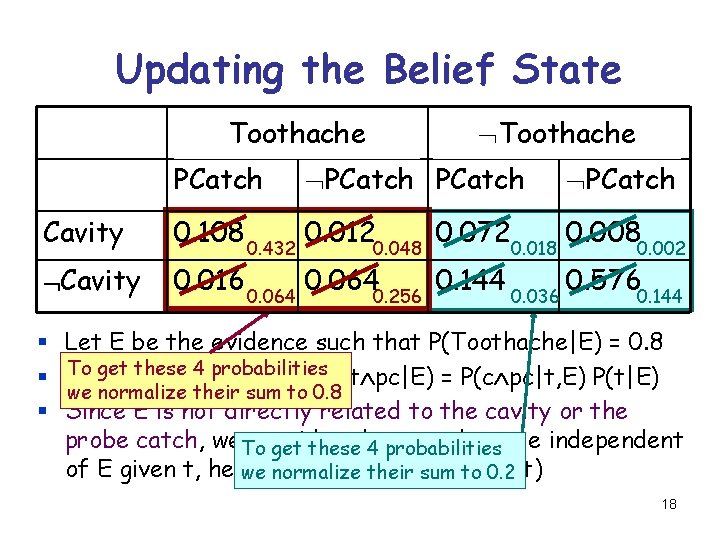Updating the Belief State Toothache PCatch Cavity § § § Toothache PCatch 0. 108 0. 432 0. 0120. 048 0. 0720. 018 0. 0080. 002 0. 016 0. 0640. 256 0. 144 0. 036 0. 5760. 144 Let E be the evidence such that P(Toothache|E) = 0. 8 To get these probabilities We want to 4 compute P(c t pc|E) = P(c pc|t, E) P(t|E) we normalize their sum to 0. 8 Since E is not directly related to the cavity or the probe catch, we. To consider that c and pc are independent get these 4 probabilities of E given t, hence: P(c pc|t, E) P(c pc|t) we normalize their = sum to 0. 2 18Issues § If a state is described by n propositions, then a belief state contains 2 n states (possibly, some have probability 0) § Modeling difficulty: many numbers must be entered in the first place § Computational issue: memory size and time 19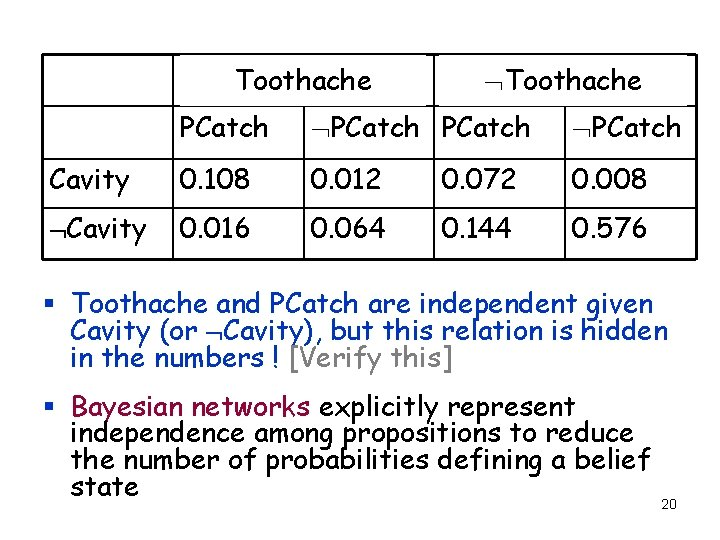Toothache PCatch Cavity 0. 108 0. 012 0. 072 0. 008 Cavity 0. 016 0. 064 0. 144 0. 576 § Toothache and PCatch are independent given Cavity (or Cavity), but this relation is hidden in the numbers ! [Verify this] § Bayesian networks explicitly represent independence among propositions to reduce the number of probabilities defining a belief state 20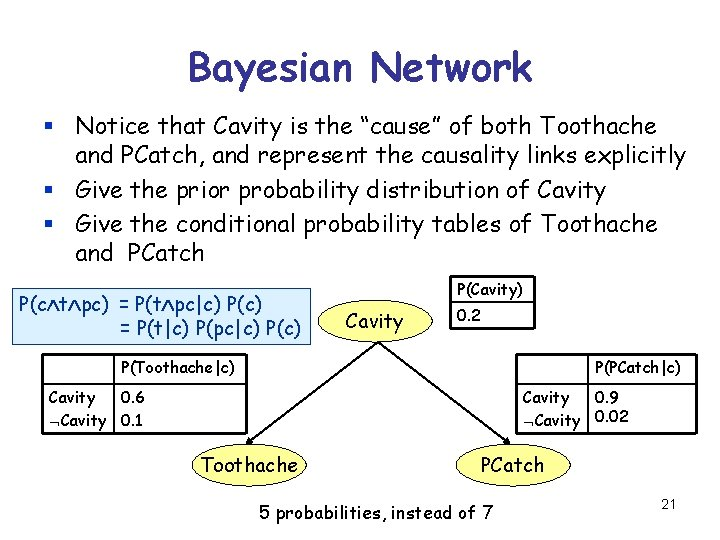Bayesian Network § Notice that Cavity is the “cause” of both Toothache and PCatch, and represent the causality links explicitly § Give the prior probability distribution of Cavity § Give the conditional probability tables of Toothache and PCatch P(c t pc) = P(t pc|c) P(c) = P(t|c) P(pc|c) P(Cavity) Cavity 0. 2 P(Toothache|c) P(PCatch|c) Cavity 0. 6 Cavity 0. 1 Cavity 0. 9 Cavity 0. 02 Toothache PCatch 5 probabilities, instead of 7 21A More Complex BN Burglary Intuitive meaning of arc from x to y: “x has direct influence on y” Earthquake causes Alarm Directed acyclic graph effects John. Calls Mary. Calls 22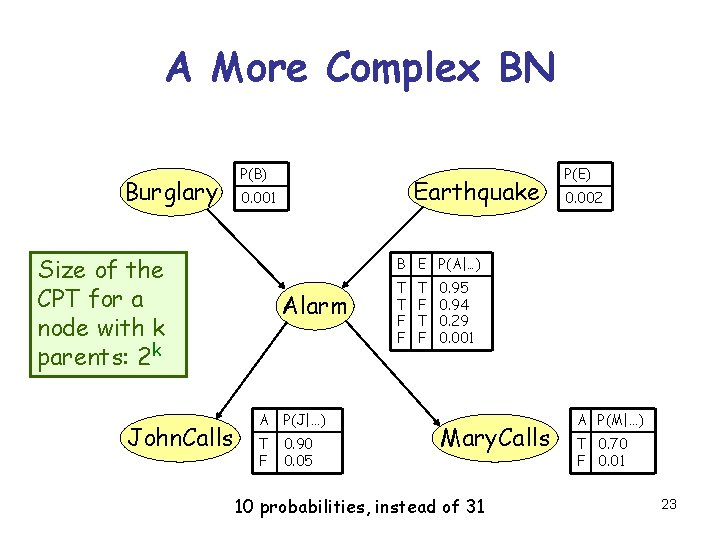A More Complex BN Burglary P(B) Size of the CPT for a node with k parents: 2 k John. Calls Earthquake 0. 001 P(E) 0. 002 B E P(A|…) Alarm A P(J|…) T F 0. 90 0. 05 T T F F T F 0. 95 0. 94 0. 29 0. 001 Mary. Calls 10 probabilities, instead of 31 A P(M|…) T 0. 70 F 0. 01 23What does the BN encode? Burglary P(b j) P(b) P(j) P(b j|a) = P(b|a) P(j|a) John. Calls Earthquake Alarm Mary. Calls Each of the beliefs John. Calls and Mary. Calls is independent of Burglary For example, John does and Earthquake given not observe any burglaries Alarm or Alarm directly 24What does the BN encode? Burglary P(b j|a) = P(b|a) P(j m|a) = P(j|a) P(m|a) John. Calls Earthquake Alarm A node is independent of Mary. Calls its non-descendants given its parents The beliefs John. Calls and Mary. Calls are For instance, the reasons why independent given John and Mary may not call if Alarm or Alarm there is an alarm are unrelated 25What does the BN encode? Burglary and Earthquake are independent John. Calls Earthquake Alarm A node is independent of Mary. Calls its non-descendants given its parents The beliefs John. Calls and Mary. Calls are For instance, the reasons why independent given John and Mary may not call if Alarm or Alarm there is an alarm are unrelated 26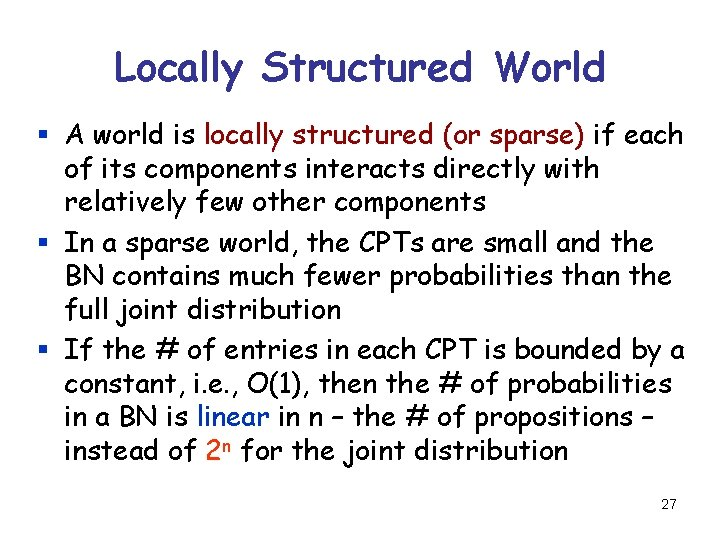Locally Structured World § A world is locally structured (or sparse) if each of its components interacts directly with relatively few other components § In a sparse world, the CPTs are small and the BN contains much fewer probabilities than the full joint distribution § If the # of entries in each CPT is bounded by a constant, i. e. , O(1), then the # of probabilities in a BN is linear in n – the # of propositions – instead of 2 n for the joint distribution 27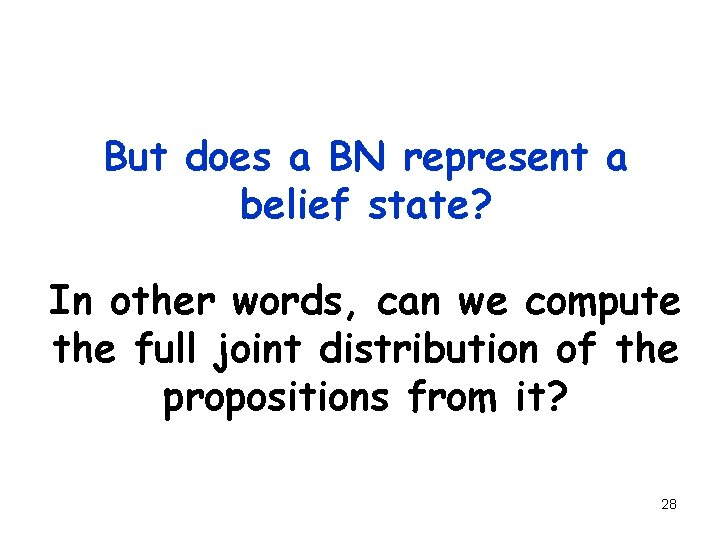But does a BN represent a belief state? In other words, can we compute the full joint distribution of the propositions from it? 28Calculation of Joint Probability Burglary P(B) Earthquake 0. 001 P(J M A B E) = ? ? Alarm John. Calls A P(J|…) T F 0. 90 0. 05 P(E) 0. 002 B E P(A|…) T T F F T F 0. 95 0. 94 0. 29 0. 001 Mary. Calls A P(M|…) T 0. 70 F 0. 01 29Burglary Earthquake Alarm John. Calls Mary. Calls § P(J M A B E) = P(J M|A, B, E) P(A B E) = P(J|A, B, E) P(M|A, B, E) P(A B E) (J and M are independent given A) § P(J|A, B, E) = P(J|A) (J and B E are independent given A) § P(M|A, B, E) = P(M|A) § P(A B E) = P(A| B, E) P( B| E) P( E) = P(A| B, E) P( B) P( E) ( B and E are independent) § P(J M A B E) = P(J|A)P(M|A)P(A| B, E)P( B)P( E) 30Calculation of Joint Probability Burglary P(B) Earthquake 0. 001 P(J M A B E) = P(J|A)P(M|A)P(A| B, E)P( B)P( E) Alarm = 0. 9 x 0. 7 x 0. 001 x 0. 999 x 0. 998 = 0. 00062 John. Calls A P(J|…) T F 0. 90 0. 05 P(E) 0. 002 B E P(A|…) T T F F T F 0. 95 0. 94 0. 29 0. 001 Mary. Calls A P(M|…) T 0. 70 F 0. 01 31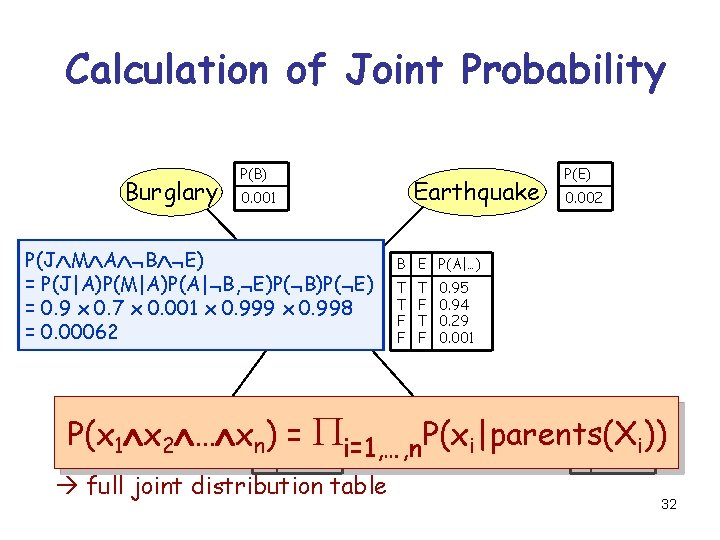Calculation of Joint Probability Burglary P(B) Earthquake 0. 001 P(J M A B E) = P(J|A)P(M|A)P(A| B, E)P( B)P( E) Alarm = 0. 9 x 0. 7 x 0. 001 x 0. 999 x 0. 998 = 0. 00062 P(E) 0. 002 B E P(A|…) T T F F T F 0. 95 0. 94 0. 29 0. 001 P(x 1 x = Pi=1, …, n. P(x John. Calls Mary. Calls T 0. 70 i)) 2 … xn. T) 0. 90 i|parents(X A P(J|…) A P(M|…) F 0. 05 F 0. 01 full joint distribution table 32Calculation of Joint Probability Since a BN defines. P(E)the Burglary 0. 001 full joint. Earthquake 0. 002 of a distribution set of propositions, it P(J M A B E) B E P(A| ) represents a belief state = P(J|A)P(M|A)P(A| B, E)P( B)P( E) T T 0. 95 P(B) … Alarm = 0. 9 x 0. 7 x 0. 001 x 0. 999 x 0. 998 = 0. 00062 T F 0. 94 F T 0. 29 F F 0. 001 P(x 1 x = Pi=1, …, n. P(x John. Calls Mary. Calls T 0. 70 i)) 2 … xn. T) 0. 90 i|parents(X A P(J|…) A P(M|…) F 0. 05 F 0. 01 full joint distribution table 33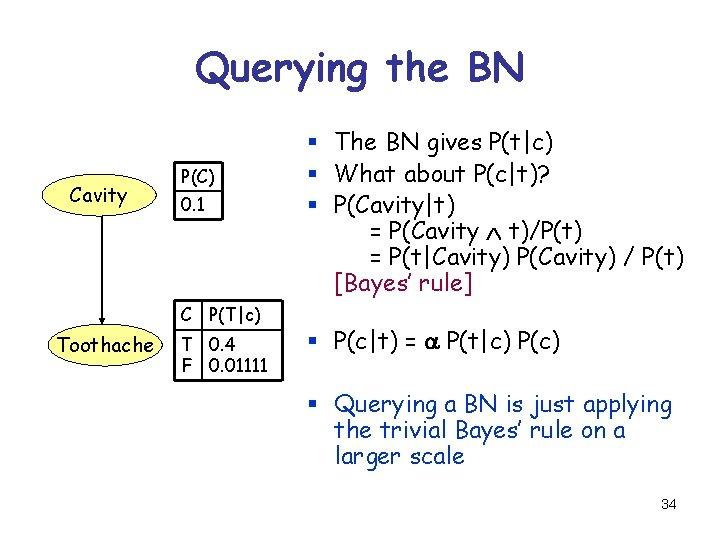Querying the BN Cavity P(C) 0. 1 C P(T|c) Toothache T 0. 4 F 0. 01111 § The BN gives P(t|c) § What about P(c|t)? § P(Cavity|t) = P(Cavity t)/P(t) = P(t|Cavity) P(Cavity) / P(t) [Bayes’ rule] § P(c|t) = a P(t|c) P(c) § Querying a BN is just applying the trivial Bayes’ rule on a larger scale 34Querying the BN § New evidence E indicates that John. Calls with some probability p § We would like to know the posterior probability of the other beliefs, e. g. P(Burglary|E) § P(B|E) = P(B J|E) + P(B J|E) = P(B|J, E) P(J|E) + P(B| J, E) P( J|E) = P(B|J) P(J|E) + P(B| J) P( J|E) = p P(B|J) + (1 -p) P(B| J) § We need to compute P(B|J) and P(B| J) 35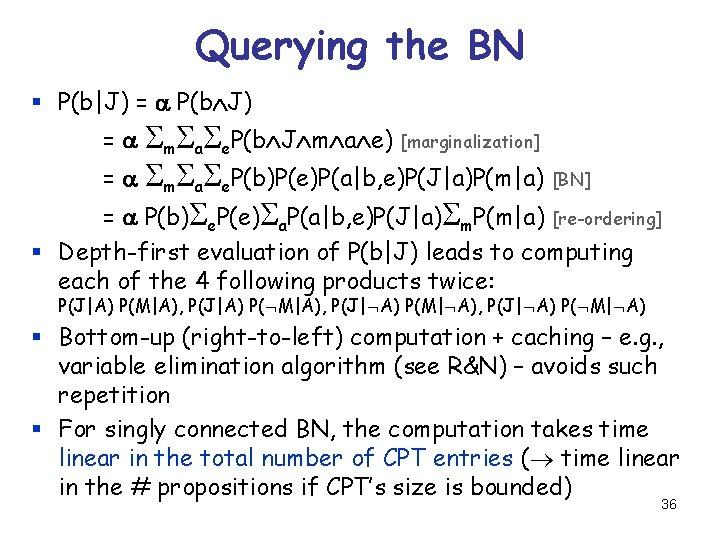Querying the BN § P(b|J) = a P(b J) = a Sm. Sa. Se. P(b J m a e) [marginalization] = a Sm. Sa. Se. P(b)P(e)P(a|b, e)P(J|a)P(m|a) [BN] = a P(b)Se. P(e)Sa. P(a|b, e)P(J|a)Sm. P(m|a) [re-ordering] § Depth-first evaluation of P(b|J) leads to computing each of the 4 following products twice: P(J|A) P(M|A), P(J|A) P( M|A), P(J| A) P(M| A), P(J| A) P( M| A) § Bottom-up (right-to-left) computation + caching – e. g. , variable elimination algorithm (see R&N) – avoids such repetition § For singly connected BN, the computation takes time linear in the total number of CPT entries ( time linear in the # propositions if CPT’s size is bounded) 36Comparison to Classical Logic Burglary Earthquake Alarm John. Calls Mary. Calls Burglary Alarm Earthquake Alarm John. Calls Alarm Mary. Calls If the agent observes John. Calls, it infers Alarm, Mary. Calls, Burglary, and Earthquake If it observes John. Calls, then it infers nothing 37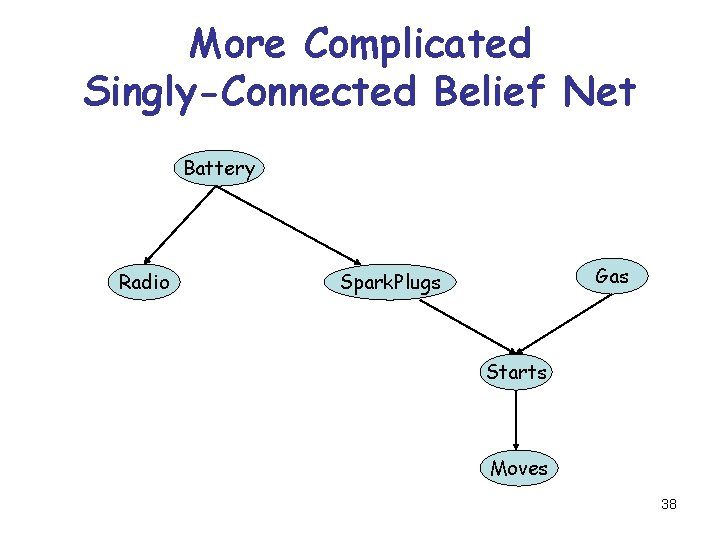More Complicated Singly-Connected Belief Net Battery Radio Gas Spark. Plugs Starts Moves 38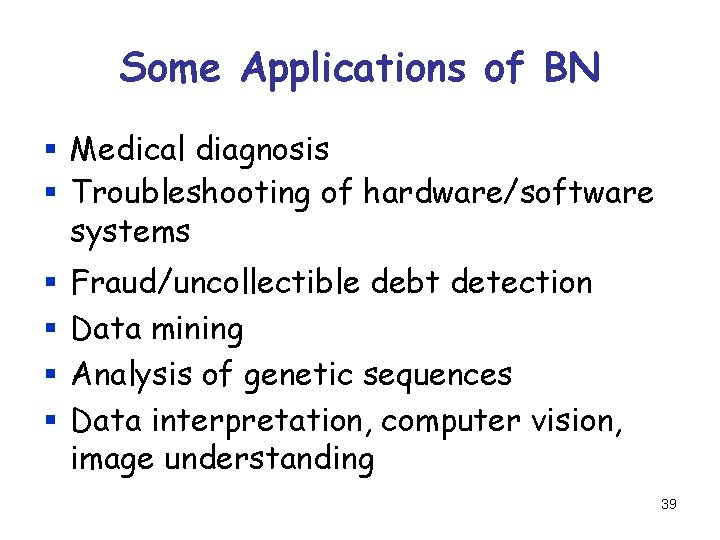Some Applications of BN § Medical diagnosis § Troubleshooting of hardware/software systems § § Fraud/uncollectible debt detection Data mining Analysis of genetic sequences Data interpretation, computer vision, image understanding 39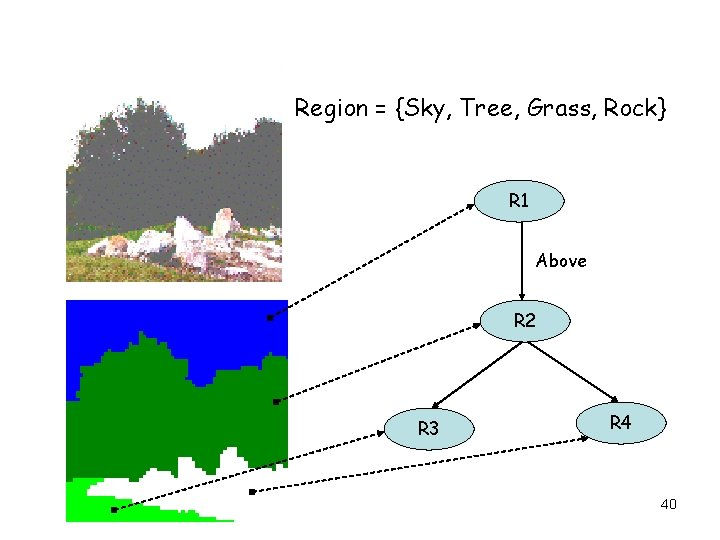Region = {Sky, Tree, Grass, Rock} R 1 Above R 2 R 3 R 4 40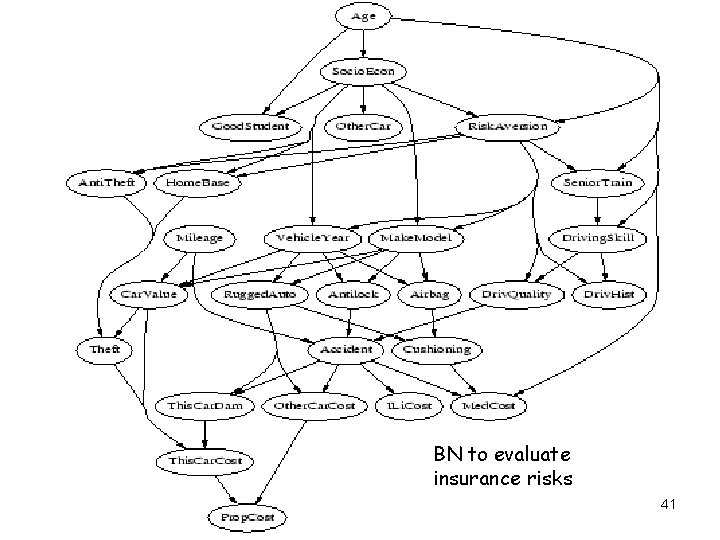BN to evaluate insurance risks 41